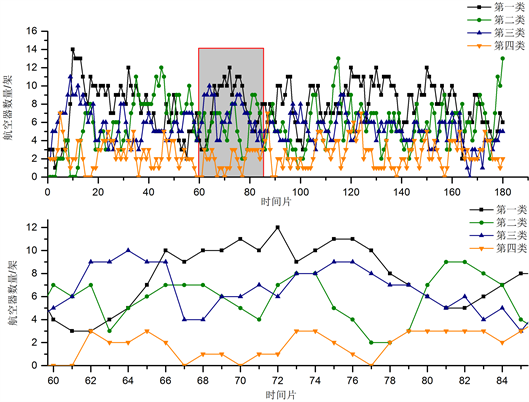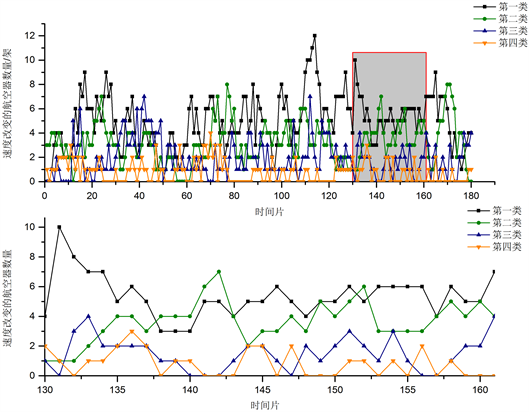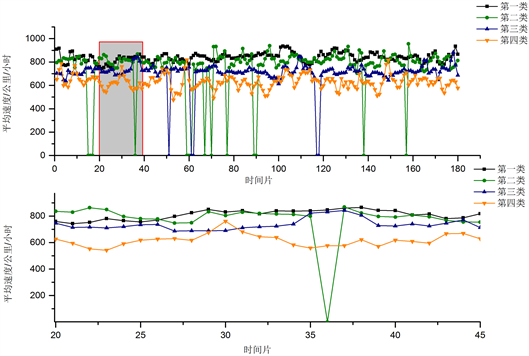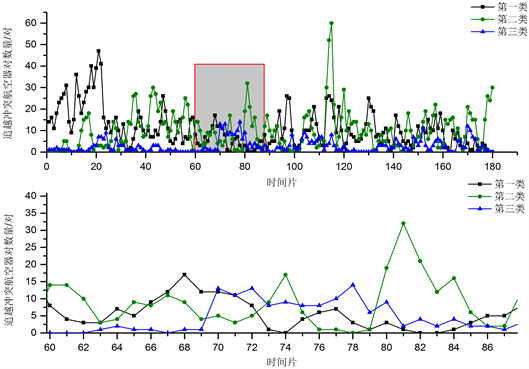﻿ 基于DTW的K-medoids扇区交通特征聚类研究

# 基于DTW的K-medoids扇区交通特征聚类研究Clustering of Sector Traffic Characteristics Based on DTW k-Medoids

Abstract: With the increasing demand for air transportation, the traffic operation mode is becoming more and more complicated, and the operation pressure of the air traffic control system is increasing day by day. How to improve the operating efficiency of the air traffic control system on the basis of meeting development needs has become a hot spot in the industry. This paper screens the traffic characteristics and behavior indicators of sectors and counts the time series of different indicators. For the high-dimensional attributes of the time series, the K-medoids clustering algorithm based on DTW was selected, and the clustering results of 15 sector samples under the traffic characteristic indicators of each sector were identified. The case analysis shows that the clustering method based on time series can identify the distribution pattern of multiple sectors under a single traffic characteristic, and finally provide an effective means and basis for the diversified analysis needs of traffic behavior.

1. 引言

2. 扇区交通行为模式指标体系

2.1. 指标体系构建

2.1.1. 密度类指标

1、航空器数量

$Nu{m}_{Sec,{t}_{k}}={\sum }_{A{c}_{Sec,{t}_{k}}^{i}\in A{c}_{Sec,{t}_{k}}}A{c}_{Sec,{t}_{k}}^{i}$ (1)

2、容流比

$Cf{r}_{Sec,{t}_{k}}=Nu{m}_{Sec,{t}_{k}}/Cp{t}_{Sec,{t}_{k}}$ (2)

3、管制里程

$Ctf{d}_{Sec,{t}_{k}}^{i}$ 表示 $A{c}_{Sec,{t}_{k}}^{i}$ 的管制飞行里程，则时段 ${t}_{k}$ 内扇区Sec中所有航班的管制飞行里程 $Ctf{d}_{Sec,{t}_{k}}$ 可具体表示为：

$Ctf{d}_{Sec,{t}_{k}}={\sum }_{i=1}^{Nu{m}_{Sec,{t}_{k}}}Ctf{d}_{Sec,{t}_{k}}^{i}$ (3)

4、管制时间

$Ctf{t}_{Sec,{t}_{k}}^{i}$ 表示 $A{c}_{Sec,{t}_{k}}^{i}$ 的管制飞行时间，则时段 ${t}_{k}$ 内扇区Sec中所有航班的管制飞行时间 $Ctf{t}_{Sec,{t}_{k}}$ 可具体表示为：

$Ctf{t}_{Sec,{t}_{k}}={\sum }_{i=1}^{Nu{m}_{Sec,{t}_{k}}}Ctf{t}_{Sec,{t}_{k}}^{i}$ (4)

2.1.2. 动态类指标

5、航向改变的航空器数量

$Ach{c}_{Sec,{t}_{k}}^{i}\in Ach{c}_{Sec,{t}_{k}}$ 表示航向改变大于15?的航空器， $Ach{c}_{Sec,{t}_{k}}$ 表示时段 ${t}_{k}$ 内扇区Sec中所有航向改变大于15?的航空器集合，则该指标 $AcH{c}_{Sec,{t}_{k}}$ 可表示为：

$AcH{c}_{Sec,{t}_{k}}={\sum }_{Ach{c}_{Sec,{t}_{k}}^{i}\in Ach{c}_{Sec,{t}_{k}}}Ach{c}_{Sec,{t}_{k}}^{i}$ (5)

6、速度改变的航空器数量

$Acs{c}_{Sec,{t}_{k}}^{i}\in Acs{c}_{Sec,{t}_{k}}$ 表示速度改变超过10节的航空器， $Acs{c}_{Sec,{t}_{k}}$ 表示时段 ${t}_{k}$ 内扇区Sec中所有速度改变大于10节的航空器集合，则该指标 $AcS{c}_{Sec,{t}_{k}}$ 可表示为：

$AcS{c}_{Sec,{t}_{k}}={\sum }_{Acs{c}_{Sec,{t}_{k}}^{i}\in Acs{c}_{Sec,{t}_{k}}}Acs{c}_{Sec,{t}_{k}}^{i}$ (6)

7、高度改变的航空器数量

$Aca{c}_{Sec,{t}_{k}}^{i}\in Aca{c}_{Sec,{t}_{k}}$ 表示高度改变超过2000英尺的航空器， $Aca{c}_{Sec,{t}_{k}}$ 表示时段 ${t}_{k}$ 内扇区Sec中所有高度改变的航空器集合，则该指标 $AcA{c}_{Sec,{t}_{k}}$ 可表示为：

$AcA{c}_{Sec,{t}_{k}}={\sum }_{Aca{c}_{Sec,{t}_{k}}^{i}\in Aca{c}_{Sec,{t}_{k}}}Aca{c}_{Sec,{t}_{k}}^{i}$ (7)

8、平均速度

$A{c}_{Sec,{t}_{k}}^{i}$ 的速度为 $Va{c}_{Sec,{t}_{k}}^{i}$，则在时段 ${t}_{k}$ 内扇区Sec中所有航空器的平均速度 ${\stackrel{¯}{V}}_{Sec,{t}_{k}}$ 可表示为：

${\stackrel{¯}{V}}_{Sec,{t}_{k}}={\sum }_{i=1}^{Nu{m}_{Sec,{t}_{k}}}Va{c}_{Sec,{t}_{k}}^{i}/Nu{m}_{Sec,{t}_{k}}$ (8)

2.1.3. 冲突类指标

9、水平最小间隔

$Minhse{p}_{Sec,{t}_{k}}=min\left[Hse{p}_{Sec,{t}_{k}}^{i,j},\left(A{c}_{Sec,{t}_{k}}^{i},A{c}_{Sec,{t}_{k}}^{j}\right)\in A{c}_{Sec,{t}_{k}}\right]$ (9)

10、垂直最小间隔

$Minvse{p}_{Sec,{t}_{k}}=min\left[Vse{p}_{Sec,{t}_{k}}^{i,j},\left(A{c}_{Sec,{t}_{k}}^{i},A{c}_{Sec,{t}_{k}}^{j}\right)\in A{c}_{Sec,{t}_{k}}\right]$ (10)

11、追越冲突航空器对数量

$Numovac{p}_{Sec,{t}_{k}}={\sum }_{\left(A{c}_{Sec,{t}_{k}}^{i},A{c}_{Sec,{t}_{k}}^{j}\right)\in A{c}_{Sec,{t}_{k}}}sign\left(Aglpai{r}_{Sec,{t}_{k}}^{i,j}\right)$ (11)

$sign\left(Aglpai{r}_{Sec,{t}_{k}}^{i,j}\right)=\left\{\begin{array}{l}1,\text{?}\text{?}\text{if}\text{?}Aglpai{r}_{Sec,{t}_{k}}^{i,j}<{15}^{°}\\ 0,\text{?}\text{?}\text{otherwise}\end{array}$ (12)

12、对头冲突航空器对数量

$Numopac{p}_{Sec,{t}_{k}}={\sum }_{\left(A{c}_{Sec,{t}_{k}}^{i},A{c}_{Sec,{t}_{k}}^{j}\right)\in A{c}_{Sec,{t}_{k}}}sign\left(Aglpai{r}_{Sec,{t}_{k}}^{i,j}\right)$ (13)

$sign\left(Aglpai{r}_{Sec,{t}_{k}}^{i,j}\right)=\left\{\begin{array}{l}1\text{?}\text{?}\text{?}\text{if}\text{?}Aglpai{r}_{Sec,{t}_{k}}^{i,j}\le {180}^{°}\vee Aglpai{r}_{Sec,{t}_{k}}^{i,j}\ge {165}^{°}\\ 0,\text{?}\text{?}\text{otherwise}\end{array}$ (14)

2.2. 交通行为模式分析方法

2.2.1. 基于DTW的K-medoids聚类算法

2.2.2. 聚类有效性评价

3. 实例验证分析

3.1. 基于单一指标的扇区交通行为聚类分析

3.1.1. 密度类指标的交通行为聚类分析Table 1. Clustering evaluation results based on the number of aircraftFigure 1. Cluster center of sector samples of the number of aircraft

3.1.2. 动态类指标的交通行为聚类分析

1、速度改变的航空器数量Table 2. Clustering evaluation results of aircraft number with speed changeFigure 2. Cluster center of sector samples of the number of aircrafts with velocity change

2、平均速度Table 3. Clustering evaluation results based on average speedFigure 3. Clustering centers of sector samples with average speed

3.1.3. 冲突类指标的交通行为聚类分析Table 4. Quantitative clustering evaluation results of aircraft pairs based on overtaking conflictFigure 4. Cluster centers of sector samples for the number of conflicting aircraft pairs

4. 总结

2020民航安全能力建设基金《机场协同决策(A-CDM)技术规范》项目支持。

 Masalonis, A., Callaham, M. and Wanke, C. (2003) Dynamic Density and Complexity Metrics for Real-Time Traffic Flow Management. 5th USA/Europe Air Traffic Management R & D Seminar, Budapest, Hungary.

 Maria, P., Luigi, P., Stephane, P., et al. (2011) Toward Air Traffic Complexity Assessment in New Generation Air Traffic Management Systems. IEEE Transactions on Intelligent Transportation Systems, 12, 809-818.
https://doi.org/10.1109/TITS.2011.2113175

 RTCA (1995) Report of the RTCA Board of Directors Select Committee on Free Flight. RTCA, Washington DC.

 Chatterji, C.B. and Sridhar, B. (2001) Measures for Air Traffic Controller Workload Prediction. 1st AIAA Aircraft, Technology, Integration, and Operations Forum, Reston, Virginia, 2.C.4-1-2.C.4-9.
https://doi.org/10.2514/6.2001-5242

 Djokic, J., Lorenz, B. and Fricke, H. (2010) Air Traffic Control Complexity as Workload Driver. Transportation Research Part C Emerging Technologies, 18, 930-936. https://doi.org/10.1016/j.trc.2010.03.005

 Vogel, M., Schelbert, K., Fricke, H., et al. (2013) Analysis of Airspace Complexity Factors’ Capability to Predict Work-Load and Safety Levels in the TMA. 10th USA/Europe Air Traffic Management R & D Seminar, Chicago, USA.

 Toy, J. (2015) Complexity Metric Comparison Study for Controller Workload Prediction in 4D Trajectory Management Environments. Delft University of Technology.

 王勇, 唐靖, 饶勤菲, 等. 高效率的K-means最佳聚类数确定算法[J]. 计算机应用, 2014, 34(5): 1331-1335.

 Kaufman, L. and Rousseeuw, P.J. (1990) Finding Groups in Data: An Introduction to Cluster Analysis. John Wiley & Sons, New York, 23-42.
https://doi.org/10.1002/9780470316801

 张艳昌. 基于信息论的时间序列聚类算法研究及应用[D]: [硕士学位论文]. 大连: 大连理工大学, 2011.

 Keogh, E. and Ratanamahatana, C.A. (2005) Exact Indexing of Dynamic Time Warping. Knowledge and Information Systems, 7, 358-386.
https://doi.org/10.1007/s10115-004-0154-9

 何晓旭. 时间序列数据挖掘若干关键问题研究[D]: [博士学位论文]. 合肥: 中国科学技术大学, 2014.

Top